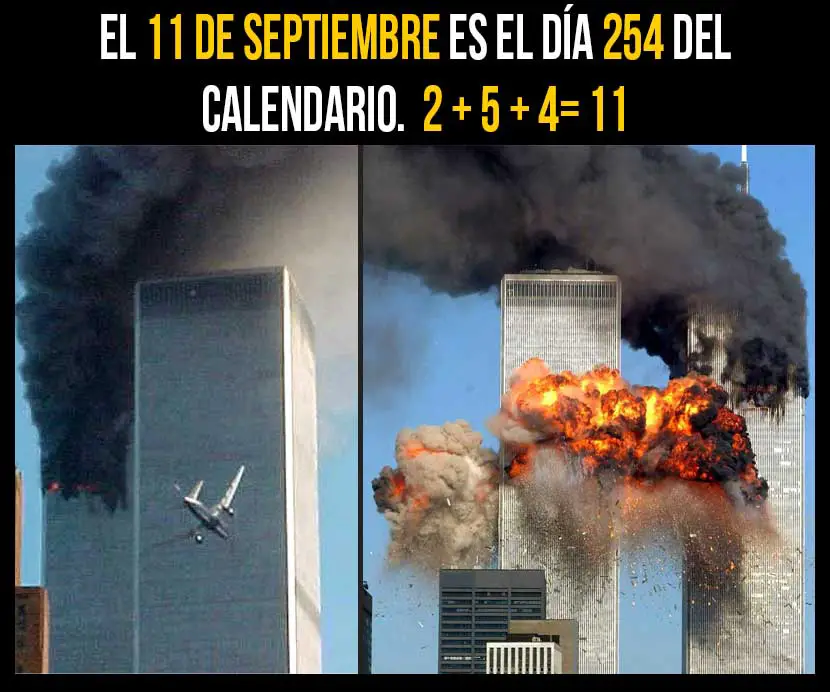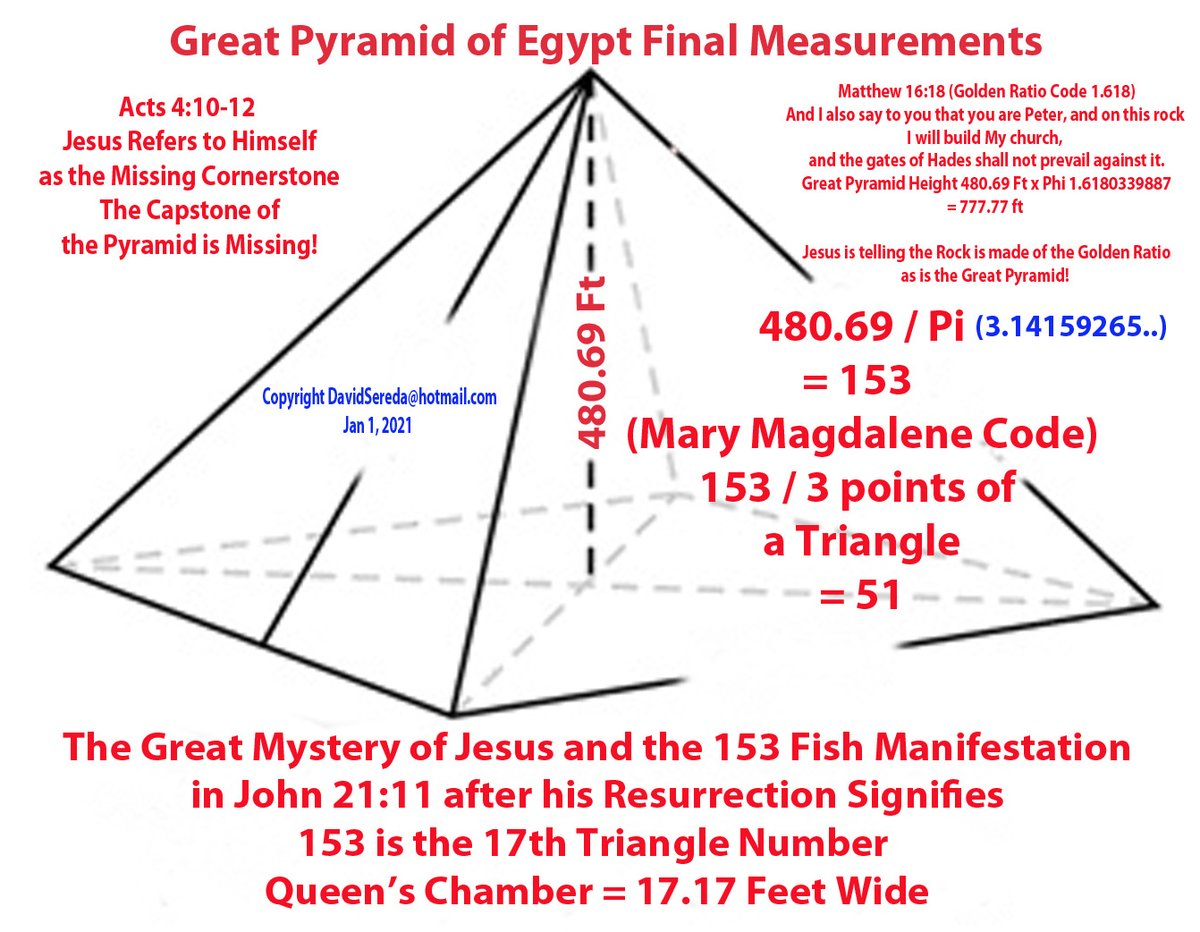Home  |  Contact

 What’s NewMessage Board
Image Gallery
Files and Documents
Polls and Test
Member List
GENERAL
REGLAS DE ESTE FORO LIBRE
Panel de quejas
CONCORDANCIAS BIBLICAS

Tools

General: FALACIA DEL HOMBRE DE PAJA ¿COMO HACE EL «PROGRE» PARA SALVAR «EL RELATO»?
Choose another message boardPrevious subject  Next subjectReply Message 1 of 8 on the subject
 From: BARILOCHENSE6999  (Original message) Sent: 22/08/2021 21:23FirstPrevious  2 to 8 of 8  NextLastReply Message 2 of 8 on the subject
 From: BARILOCHENSE6999 Sent: 22/08/2021 22:25

 Reply Message 3 of 8 on the subject
 From: BARILOCHENSE6999 Sent: 22/08/2021 23:46

 Reply Message 4 of 8 on the subject
 From: BARILOCHENSE6999 Sent: 17/09/2021 00:35The amount of days in a solar year plus the proportions for the Equatorial circumference of the Earth and the proportions of the Great Pyramid of Giza according to Golden Pi = 4/√φ = 3.144605511029693144: The Great Pyramid of Giza is a geodetic model of Planet Earth. The measurements mentioned below are the ideal measurements. A meter is equal to 100 centimeters 1 Solon cubit = 40 times √φ = 50.88078598056276 centimeters. If 1 Solon cubit is divided into 20 equal units of measure then 20 inches can be derived because 1 Solon cubit is equal to 20 inches. 1 Saylen cubit = 50 times √φ = 63.60098247570345 centimeters. If 1 Saylen cubit is divided into 25 equal units of measure then 25 inches can be derived because 1 Saylen cubit is equal to 25 inches. 1 inch = 2 times √φ = 2.544039299028138 centimeters. 1 foot = 12 inches. 1 foot = 24 times √φ = 30.528471588337656 centimeters. If the shortest edge length of a Kepler right triangle is equal to 1 then the hypotenuse of the Kepelr right triangle is equal to The Golden ratio = (√(5) plus 1)/2 = φ = 1.618033988749895 according to the Pythagorean theorem. The Golden ratio in Trigonometry = (cosine (36 degrees) times 2) = 1.618033988749895. If the shortest edge length of a Kepler right triangle is equal to 1 then second longest edge length of the Kepelr right triangle is the square root of the Golden ratio = √φ = 1.272019649514069 according to the Pythagorean theorem. The width for the square base of the Great Pyramid of Giza is equal to 756 feet. • If the shortest edge length of a Kepler right triangle is equal to 1 foot and then the second edge length of the Kepler right triangle is multiplied 378 equal times the result will be the height of the Great Pyramid of Giza = 378 times √φ = 480.823427516318082 feet according to Golden Pi = 4/√φ = 3.144605511029693144. • If the shortest edge length of a Kepler right triangle is equal to 1 foot and then the hypotenuse of the Kepler right triangle is multiplied 378 equal times the result will be the slant height of the Great Pyramid of Giza = 378 times φ = 611.61684774746031 feet according to Golden Pi = 4/√φ = 3.144605511029693144. • If the shorter edge length of a Golden ratio = (√(5) plus 1)/2 = φ = 1.618033988749895 rectangle is equal to 1 foot and then the diagonal of the Golden ratio = (√(5) plus 1)/2 = φ = 1.618033988749895 rectangle is multiplied 378 equal times the result will be the edge height of the Great Pyramid of Giza = 378 times = Cosine (18) degrees times 2 = 1.902113032590307 = 718.998726319136046 feet according to Golden Pi = 4/√φ = 3.144605511029693144.Cosine (18) degrees times 2 = 1.902113032590307. Cosine (18) degrees times 2 = 1.902113032590307 squared = φ plus 2 = 3.618033988749895. • If the shortest edge length of a Kepler right triangle is equal to 1 foot = 24 times √φ = 30.528471588337656 centimeters then the second longest edge length of that Kepler right triangle is equal to 38.832815729997479 centimeters.24 times √φ = 30.528471588337656 centimeters times the square root of the Golden ratio = √φ = 1.272019649514069 = 38.832815729997479 centimeters. 1 foot = 24 times √φ = 30.528471588337656 centimeters times the square root of the Golden ratio = √φ = 1.272019649514069 = 38.832815729997479 centimeters times 378 = the height of the Great Pyramid of Giza of Giza = 14678.804345939047062 centimeters. The height of the Great Pyramid of Giza = 14678.804345939047062 centimeters divided by 24 times √φ = 30.528471588337656 centimeters = the height of the Great Pyramid of Giza = 480.823427516318088 feet. 378 times √φ = 1.272019649514069 = 480.823427516318088. • If the shortest edge length of a Kepler right triangle is equal to 1 foot = 24 times √φ = 30.528471588337656 centimeters then the hypotenuse of that Kepler right triangle is equal to 49.39610465451582 centimeters. 24 times √φ = 30.528471588337656 centimeters times the Golden ratio = (√(5) plus 1)/2 = φ = 1.618033988749895 = 49.39610465451582 centimeters. 1 foot = 24 times √φ = 30.528471588337656 centimeters times the Golden ratio = (√(5) plus 1)/2 = φ = 1.618033988749895 378 = 49.39610465451582 centimeters times 378 = slant the height of the Great Pyramid of Giza = 18671.727559406979971centimeters. The Slant height of the Great Pyramid = 18671.727559406979971 centimeters divided by 24 times √φ = 30.528471588337656 centimeters = the slant height of the Great Pyramid of Giza = 611.61684774746031 feet. 378 times √φ = 1.272019649514069 = 611.61684774746031. • If the shortest edge length of a Golden ratio = (√(5) plus 1)/2 = φ = 1.618033988749895 rectangle is equal to 1 foot = 24 times √φ = 30.528471588337656 centimeters then the length of the diagonal of that Golden ratio = (√(5) plus 1)/2 = φ = 1.618033988749895 rectangle is equal to 58.068603673239965 centimeters. 24 times √φ = 30.528471588337656 centimeters times Cosine (18) degrees times 2 = 1.902113032590307 = 58.068603673239965 centimeters. Cosine (18) degrees times 2 = 1.902113032590307 squared = φ plus 2 = 3.618033988749895. 1 foot = 24 times √φ = 30.528471588337656 centimeters times = Cosine (18) degrees times 2 = 1.902113032590307 = 58.068603673239965 centimeters times 378 = the edge height of the Great Pyramid of Giza of Giza = 21949.932188484706835 centimeters. The edge height of the Great Pyramid = 21949.932188484706835 centimeters divided by 24 times √φ = 30.528471588337656 centimeters = the edge height of the Great Pyramid of Giza = 718.998726319136046 feet. 378 times Cosine (18) degrees times 2 = 1.902113032590307 = 718.998726319136046. Cosine (18) degrees times 2 = 1.902113032590307. Cosine (18) degrees times 2 = 1.902113032590307 squared = φ plus 2 = 3.618033988749895. A Kepler right triangle can be created from the construction of a Golden ratio = (√(5) plus 1)/2 = φ = 1.618033988749895 rectangle by using Compass and straight edge and obviously a marker for the drawing surface. The amount of days in a Solar year = 4/√φ times 7920 times 5280/(10 ^ 3 times 360) = 365.277376161209156. The amount of days in a Solar year = 4/√φ = 3.144605511029693144 times 7920 times 5280/(10 ^ 3 times 360) = 365.277376161209156. The equatorial circumference of planet Earth = 10 ^ 3 times 360 times 365.277376161209156 = 131499855.41803529616 feet. The equatorial circumference of planet Earth = 4/√φ = 3.144605511029693144 times 7920 = 24905.275647355169727 statute miles. 131499855.41803529616 feet divided by 86400 = half the perimeter of the socle of the Great Pyramid of Giza = 1521.989067338371483 feet. Half the perimeter of the socle of the Great Pyramid of Giza times 86400 is also equal to the equatorial circumference of planet Earth = 131499855.41803529616 feet. 484 divided by √φ times 2 = the width of the socle of the Great Pyramid of Giza = 760.99453366918572 feet. Half the width of the socle of the Great Pyramid of Giza = 380.49726683459286 feet times √φ = 484 feet. 484/√φ times 2 times 2 times 86400 = 131499855.41803529616 feet. 131499855.41803529616 feet divided by 5280 = The perimeter of the socle of the Great Pyramid of Giza = 484/√φ times 8 = 3043.978134676742966 feet. 484 feet /√φ times 8 = 3043.978134676742966 feet times 12 = 24905.275647355169727 statute miles. 24905.275647355169727 statute miles divided by 7920 statute miles = Golden Pi = 4/√φ = 3.144605511029693144. There are 929.28 meters in the square perimeter of the socle of the Great Pyramid of Giza according to Golden Pi = 4/√φ = 3.144605511029693144. 484/√φ times 8 = 3043.978134676742966 times 12 = 36527.737616120915592 divided by 100 = the exact amount of days in a solar year = 365.277376161209156. The equatorial diameter of our planet Earth = 41817600 feet. 41817600 feet divided 86400 = the height of the Great Pyramid of Giza = 378 times √φ = 480.823427516318082 feet plus the height of the socle of the Great Pyramid of Giza = 3.176572483681918 feet. 10 ^ 3 times 360 times 484/(√φ) times 8 times 12/(100) = 131499855.41803529616 feet. The height of the Great Pyramid if Giza is 378 times √φ = 480.823427516318082 feet. The width of the square base of the Great Pyramid of Giza is 756 feet. The perimeter of the square base of the Great Pyramid of Giza = 3024 feet. 9 factorial = 362880. At 10 degrees latitude the length of a degree is 9 factorial =362880 feet. The amount of inches in the perimeter of the square of the Great Pyramid of Giza = 36288. 36288 times 10 = 362880. The width for the square base of the Great Pyramid of Giza = 756 feet. The perimeter of the square base of the Great Pyramid of Giza = 3024 feet. There are 36288 inches in the perimeter of the square base of the Great Pyramid of Giza. 3024 times 12 = 36288. 756 times 4 times 12 = 36288. (9!)/10 = 36288. The equatorial circumference of planet Earth: https://joedubs.com/four-earthly-elements/equatorial-circumference-of-earth/ Kepler right triangle diagram with squares upon the edges of the Kepler right triangle: https://drive.google.com/file/d/1iBtXYy06yv9UWtGP5mwMXyt80vaysFvR/view?usp=sharing Kepler right triangle construction method: https://drive.google.com/file/d/15DNXB_xNP2f2jCroC0FyNUyBYGhVAA2J/view?usp=sharing PYTHAGOREAN THEOREM: https://en.wikipedia.org/wiki/Pythagorean_theorem Golden ratio: https://en.wikipedia.org/wiki/Golden_ratio The history of the meter: The history of the meter: https://www.factinate.com/editorial/meter-history/ The meter: https://en.wikipedia.org/wiki/Metre The meter is based now on the speed of light: https://www.youtube.com/watch?v=vgqUyFaUDcI

 Respuesta Mensaje 13 de 14 en el temaRespuesta Mensaje 14 de 14 en el temaReply Message 5 of 8 on the subject
 From: BARILOCHENSE6999 Sent: 12/10/2021 02:13Reply Message 6 of 8 on the subject
 From: BARILOCHENSE6999 Sent: 19/10/2021 21:02

 Reply Message 7 of 8 on the subject
 From: BARILOCHENSE6999 Sent: 24/10/2021 13:27

 Reply Message 8 of 8 on the subject
 From: BARILOCHENSE6999 Sent: 31/10/2021 02:58FirstPrevious  2 a 8 de 8  NextLastPrevious subject  Next subject